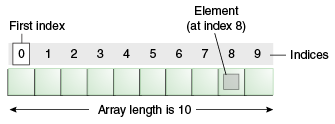# Collection Part-1 : Java Arrays

#### Posted on July 28, 2016 by By Nikhilesh, in ETL, Java, Javascript | 0

Arrays are objects which store multiple variables of the same type, it is a collection of similar type of elements that have contiguous memory location.

The length of an array is established when the array is created. After creation, its length is fixed.An array of 10 elements.

It is an array of size 10, means we can store 10-elements in a single variable/object.

Define an array:

int[] arr = new int;

int[] arr ={1,2,3,4,5};

//We can give any number here. It will allocate 10 int-variable space in JVM heap section.

Starting with arr=10;

to

arr[arr.length -1] =100; // arr.length -1=9 , because we are starting from 0 index.

How to copy one array content to another array,

public static void arraycopy( Object sourceArray, int sourceArrayPositionPos, Object destinationArray, int destinationArrayPosition, int length )

src — This is the source array.

srcPos — This is the starting position in the source array.

dest — This is the destination array.

destPos — This is the starting position in the destination data.

length — This is the number of array elements to be copied.

Use this function to copy one array into another . Ex: //here declaration, instantiation and initialization all are done at a time int[] arr ={8,7,6,5,4,3,2,2,0}; //Assume it is a sourceArray. int[] FinalArr = new int; //Assume it is a destination array , where you want to copy your content. So copy command is:

System.arraycopy(arr, 0, FinalArr, 0, arr.length); //to print this destination/Targeted array.

for(int i=0;i<arr.length;i++)

{ System.out.println(“Value-”+i+” are : ”+FinalArr[i]); }

O/P

Value-0 are : 8

Value-1 are : 7

Value-2 are : 6

Value-3 are : 5

Value-4 are : 4

Value-5 are : 3

Value-6 are : 2

Value-7 are : 2

Value-8 are : 0

EX:

int c1[][][][]={{{{1,3,4},{3,4,5},{1,2,3}},{{1,3,4},{3,4,5},{1,2,3}},{{1,3,4},{3,4,5},{1,2,3}}},{{{1,3,4},{3,4,5},{1,2,3}},{{1,3,4},{3,4,5},{1,2,3}},{{1,3,4},{3,4,5},{1,2,3}}}};

for(int i=0;i<2;i++){

for(int j=0;j<3;j++){

for(int k=0;k<3;k++){

for(int l=0;l<3;l++){

System.out.print(c1[i][j][k][l]+””);

}

}

System.out.print(“\t”);

}

System.out.print(“\n”);

}

O/P

134345123 134345123 134345123
134345123 134345123 134345123

Enjoy learning….   🙂Best Open Source Business Intelligence Software Helical Insight is Here